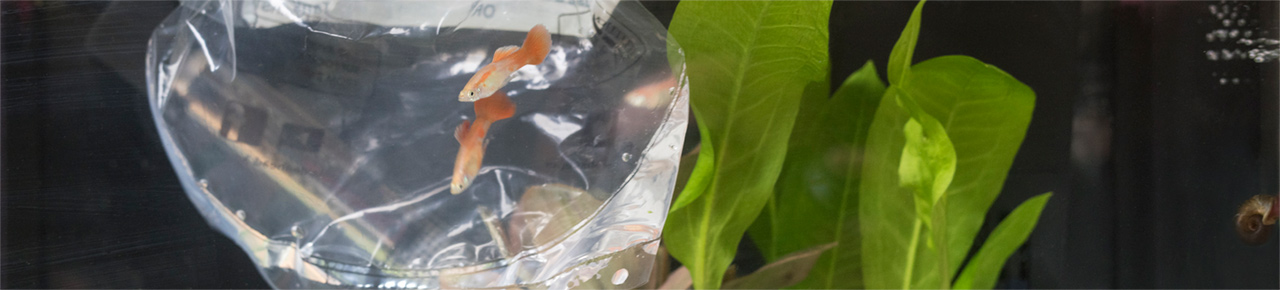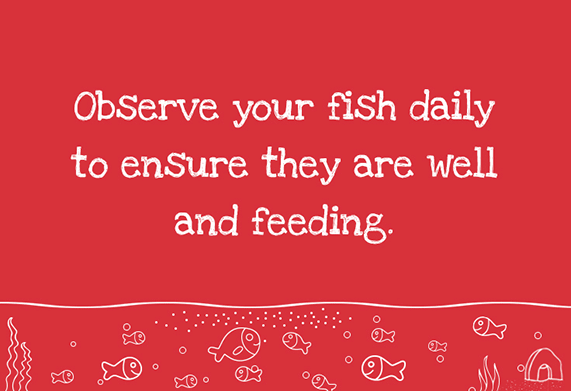# Fish keeping FAQsHow do I calculate the volume of my aquarium?

The length x depth x width in metres, multiplied by 1000 will give the volume in litres.

To work this out you might need to convert inches to cm, the equation for this is 1inch = 2.54cm. For example 12 inches x 2.54 = 30.48cm. 30.48 / 100 = size in metres.

If you then want to convert the litres to US Gallons, multiply the volume in litres by 0.264.

For example: 20 litre aquarium 20 x 0.264 = 5.28 US Gallons

Should I drain my aquarium?
What is the Nitrogen cycle?
What is New Tank Syndrome/New Aquarium Syndrome?
What is 'cycling' a fish tank?
How many fish can I keep in my aquarium?
Why has the water gone cloudy?
Why has the water gone green?
Why are my fish sick?
My fish is flicking on the ornaments, what's wrong?
Why is my fish jumping?
Should I remove the sick fish from the aquarium?
What do I do with dead fish?